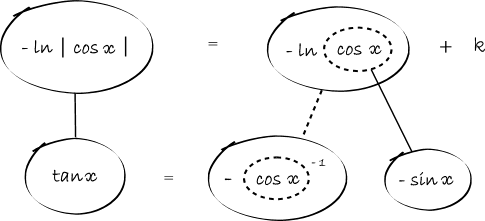1. ## Differentiating

I want to integrate -ln|cos(x)|+k, I and I should end with tan(x).

However, I'm stuck. I don't know what I need to do after $\displaystyle -x^{-1}*|cos(x)|-ln(x)*sin(x)$

This is how far I've gotten so far:2. Hello

Something I do not understand : the title of your post is "differentiating" and you want to integrate -ln|cos(x)|+k ?

I think that you want to differentiate -ln|cos(x)|+k
Differentiating ln(u(x)) gives u'(x) / u(x)
Here it gives sin(x)/cos(x) = tan(x)

3. Hello,Originally Posted by No Logic SenseI want to integrate -ln|cos(x)|+k, I and I should end with tan(x).

However, I'm stuck. I don't know what I need to do after $\displaystyle -x^{-1}*|cos(x)|-ln(x)*sin(x)$

This is how far I've gotten so far:I think you have a little problem with the chain rule of differentiation.

It is stated as below :

$\displaystyle [f(g(x))]'=g'(x)f'(g(x))$

To let you visualize it, if you let $\displaystyle t=g(x)$, it'll be :
$\displaystyle [f(t)]'=g'(x)f'(t)$

So here, what do you have ?
$\displaystyle \ln |\cos(x)|$

so $\displaystyle f(t)=\ln |\cos(x)|$

so f is the logarithm function.
and $\displaystyle t=g(x)=\cos(x)$

by using the formula, you'll have the derivative of your function :

$\displaystyle =\underbrace{(-\sin(x))}_{g'(x)} \cdot \overbrace{\frac{1}{t}}^{f'(t)}=-\sin(x) \frac{1}{\cos(x)}$

it's not formal, but it helps you understand how to use the formula.

Note that you just have to multiply the final result by -1 to get the derivative you're looking for

4. Visual AND formal...As usual, straight continuous lines differentiate with respect to x and the straight dashed line with respect to the dashed balloon expression, so that the triangular network satisfies the chain rule.

Don't integrate - balloontegrate! Balloon Calculus: worked examples from past papers

#### Search Tags

differentiating